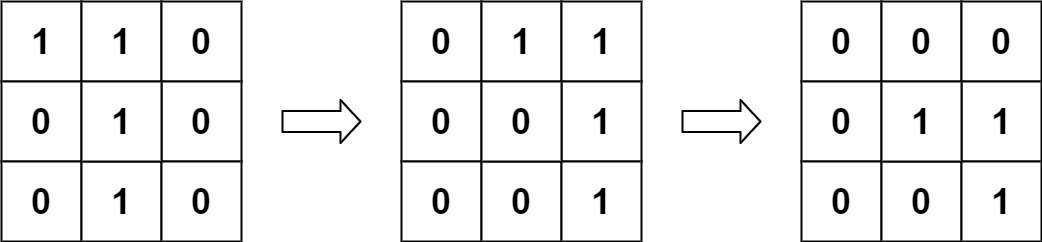# LeetCode #835 – Image Overlap

Hello, coding enthusiasts! Today, we’re diving into an intriguing problem from LeetCode called “Image Overlap”.

This problem presents us with two binary images, represented as 2D matrices, and asks us to find the maximum overlap between the two. Sounds exciting, right? Let’s get started!

## Problem Statement

You are given two images, `img1` and `img2`, represented as binary, square matrices of size `n x n`. A binary matrix has only `0`s and `1`s as values.

We translate one image however we choose by sliding all the `1` bits left, right, up, and/or down any number of units. We then place it on top of the other image. We can then calculate the overlap by counting the number of positions that have a `1` in both images.

Note also that a translation does not include any kind of rotation. Any `1` bits that are translated outside of the matrix borders are erased.

Return the largest possible overlap.

Example 1:

``````Input: img1 = [[1,1,0],[0,1,0],[0,1,0]], img2 = [[0,0,0],[0,1,1],[0,0,1]]
Output: 3
Explanation: We translate img1 to right by 1 unit and down by 1 unit.``````The number of positions that have a 1 in both images is 3 (shown in red).Example 2:

``````Input: img1 = [], img2 = []
Output: 1``````

Example 3:

``````Input: img1 = [], img2 = []
Output: 0``````

## Constraints:

• `n == img1.length == img1[i].length`
• `n == img2.length == img2[i].length`
• `1 <= n <= 30`
• `img1[i][j]` is either `0` or `1`.
• `img2[i][j]` is either `0` or `1`.

## Analysis

The solution to this problem involves a technique called convolution, a mathematical operation that provides a measure of the overlap between two matrices. In our case, we’ll use convolution to determine the maximum overlap between the two binary images.

## Approach

Here’s a step-by-step breakdown of our approach:

1. Identify the ‘1’ coordinates: First, we find all the coordinates in both images where the pixel value is 1.
2. Calculate relative positions: Next, we calculate the relative positions for each pair of 1-coordinates between `A` and `B`. The relative position is simply the difference between the x and y coordinates of the 1-pixel in `A` and the corresponding 1-pixel in `B`.
3. Count the frequencies: We then count the frequency of each relative position using a hash map. The relative position with the highest frequency will give us the maximum overlap.
4. Return the maximum overlap: Finally, we return the maximum count from the hash map, which represents the maximum overlap of 1s between `A` and `B`.

## Time Complexity

The time complexity of this solution is O(N^4), where N is the size of the matrices. This is because we are iterating over all the elements of the matrices for each pair of 1-coordinates.

## Space Complexity

The space complexity is O(N^2), as we are storing all the 1-coordinates from both matrices and the relative positions in a hash map.

## Code

### Python

Python
``````class Solution:
def largestOverlap(self, A: List[List[int]], B: List[List[int]]) -> int:
N = len(A)
LA = [(i, j) for i in range(N) for j in range(N) if A[i][j]]
LB = [(i, j) for i in range(N) for j in range(N) if B[i][j]]
c = collections.Counter((x1 - x2, y1 - y2) for (x1, y1) in LA for (x2, y2) in LB)
return max(c.values() or )``````

### JavaScript

JavaScript
``````var largestOverlap = function(A, B) {
let N = A.length;
let LA = [], LB = [];
for(let i = 0; i < N; i++) {
for(let j = 0; j < N; j++) {
if(A[i][j]) LA.push([i, j]);
if(B[i][j]) LB.push([i, j]);
}
}
let c = new Map();
for(let [x1, y1] of LA) {
for(let [x2, y2] of LB) {
let key = `\${x1 - x2},\${y1 - y2}`;
c.set(key, (c.get(key) || 0) + 1);
}
}
let maxOverlap = 0;
for(let val of c.values()) {
maxOverlap = Math.max(maxOverlap, val);
}
return maxOverlap;
};``````

## Conclusion

And there you have it! We’ve successfully solved the “Image Overlap” problem from LeetCode. This problem is a great example of how we can use mathematical techniques like convolution in programming to solve interesting problems.

Happy coding!